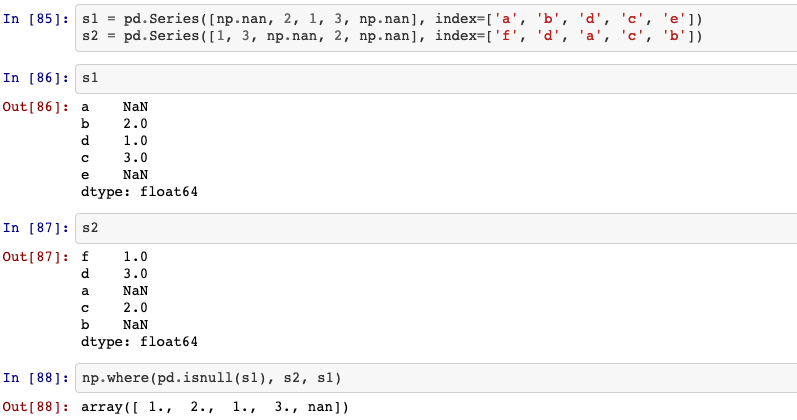# 24 things to know about pandas series

24 things to know about pandas series

To get start with pandas, you need to get confortable with its two data structures: series and dataframe. In this blog, we will talk about multiple applications of series.

## 1. Creating a series

pandas.Series(data=None, index=None, dtype=None, name=None, copy=False, fastpath=False)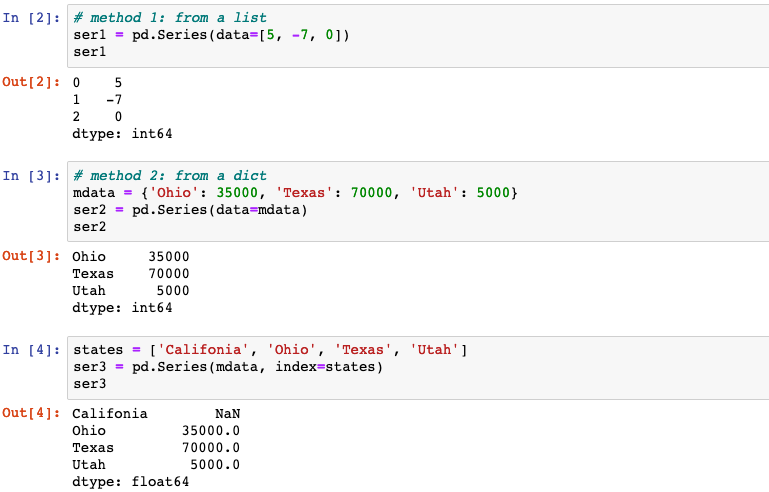## 2. index

Series.index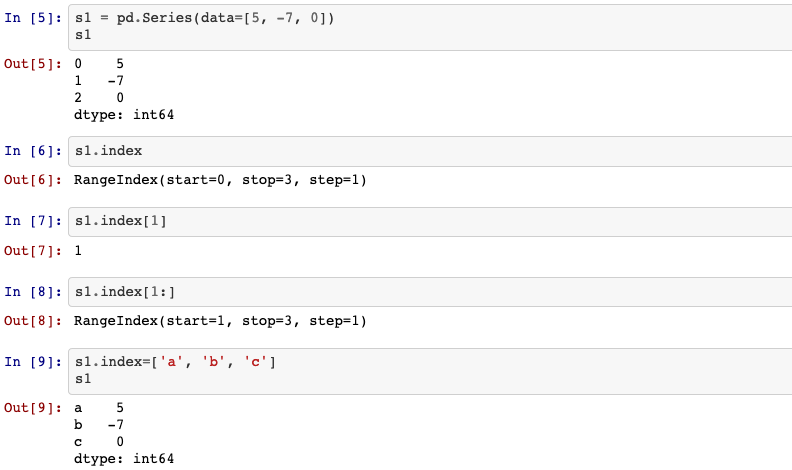## 3. Indexing & selection

Series indexing (ser2[...]) works analogously to NumPy array indexing, except you can use the Series’s index values instead of only integers.

Moreover, you can also select a subset of the rows from a series with NumPy-like notation using either axis labels (loc) or integers (iloc).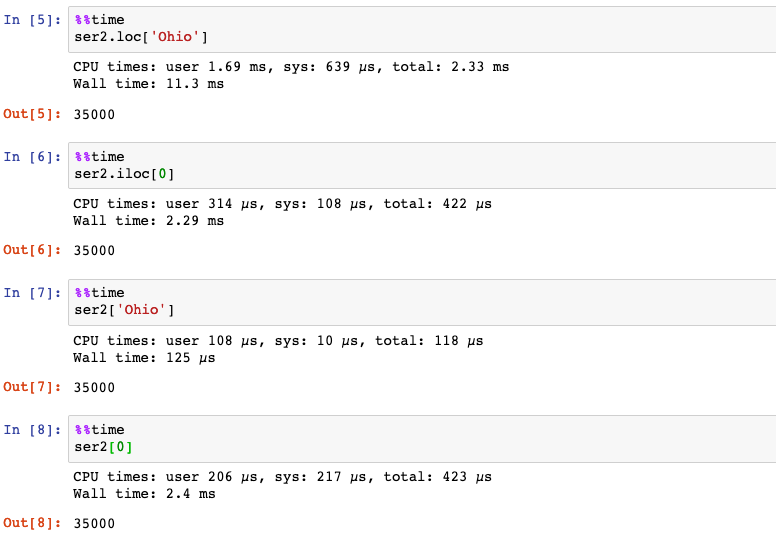The selection syntax ser2[:2] is provided as a convenience. Passing a single element or a list to the [] operator selects columns.

Slicing with labels like ser2['Ohio':'Texas'] behaves differently than normal Python slicing in that the end-point is inclusive.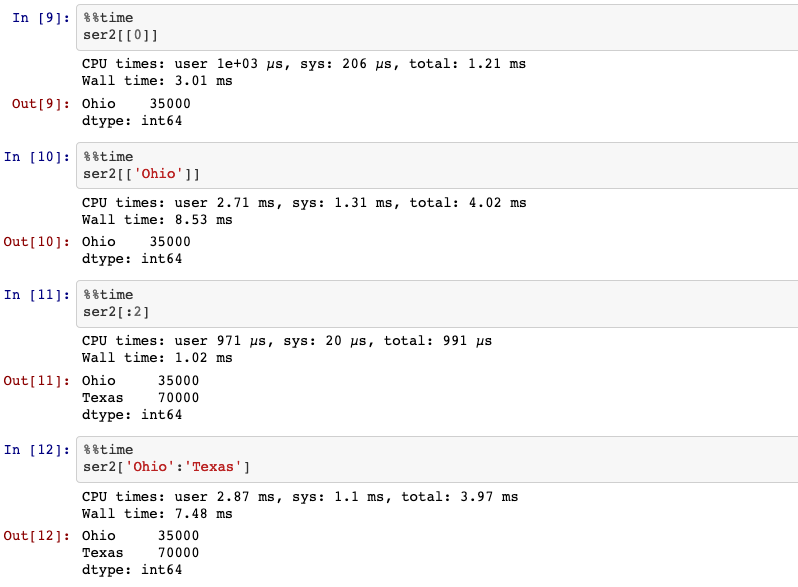## 4. Hierarchical indexing

Hierarchical indexing is an important feature of pandas that enables you to have multiple (two or more) index levels on an axis. It provides a way for working with higher dimensional data in a lower dimensional form.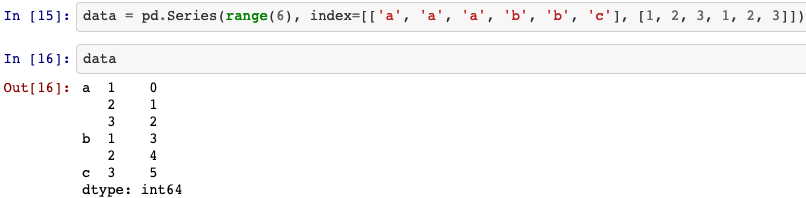With a hierarchically indexed object, so-called partial indexing is possible, enabling you to concisely select subsets of the data: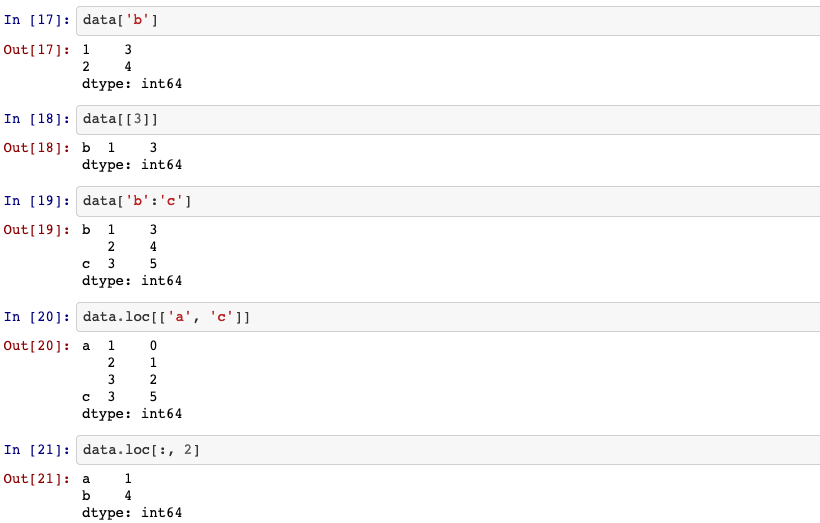Hierarchical indexing plays an important role in reshaping data and group-based operations like forming a pivot table. For instance, you can rearrange the dataset into a dataframe with unstack method: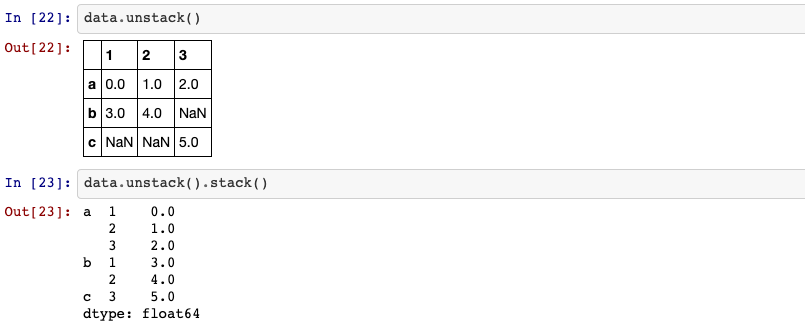## 5. reindex

An important method on pandas objects is reindex, which means to create a new object with the data consormed to a new index.

Series.reindex(self, index=None, **kwargs)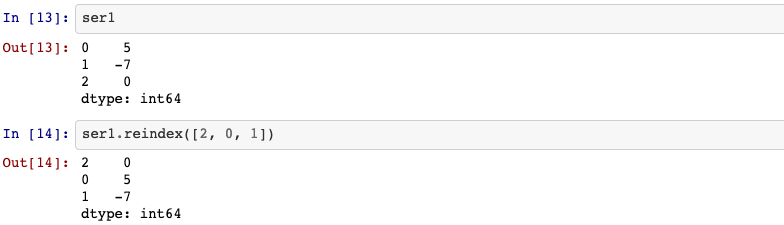## 6. drop

drop method returns a new object with the indicated value or values deleted from an axis.

Series.drop(self, labels=None, axis=0, index=None, columns=None, level=None, inplace=False, errors='raise')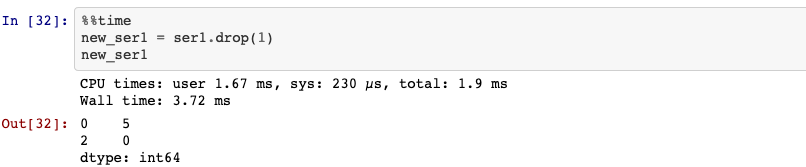## 7. Arithmetic

Using NumPy functions or NumPy-like operations, such as filtering with a boolean array, scalar multiplication, or applying math functions, will preserve the index-value link: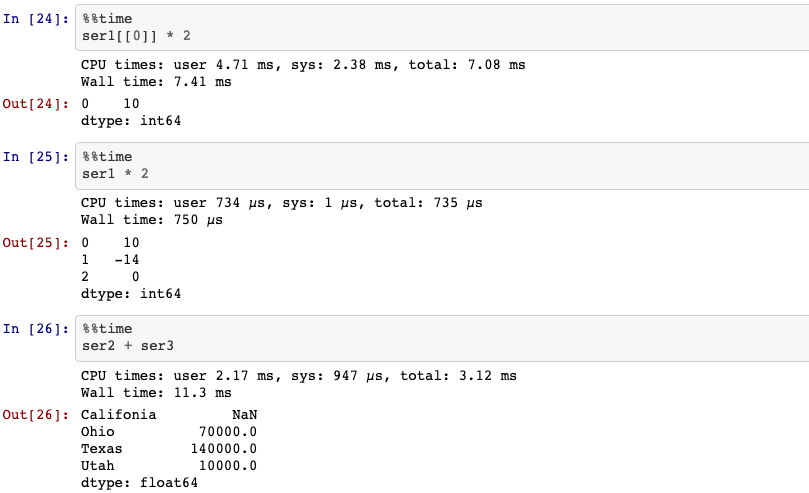When you are adding together objects, if any index pairs are not the same, the respective index in the result will be the union of the index pairs.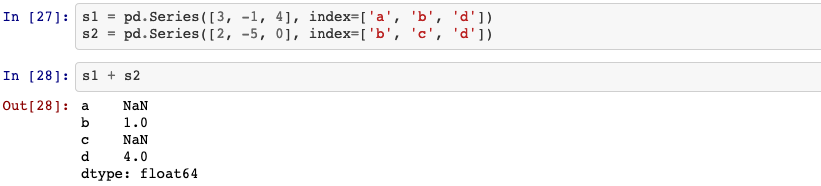## 8. in

To see if the value is one of the series’ index.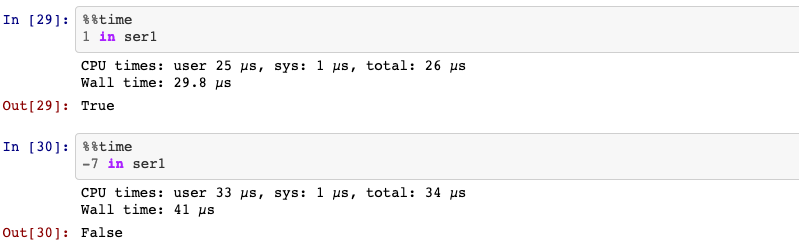## 9. isnull

Detect missing values for an array-like object.

pandas.isnull(obj)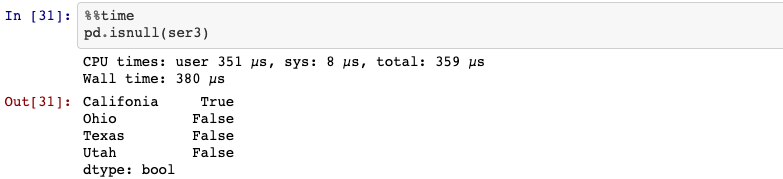## 10. sorting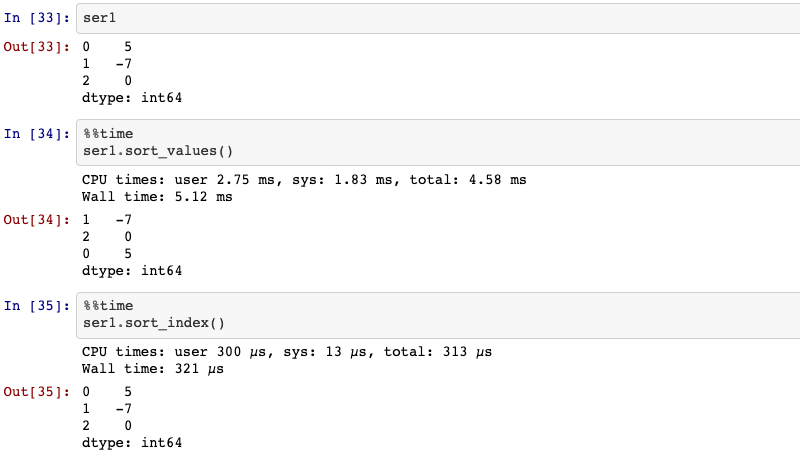## 11. ranking

Compute numerical data ranks (1 through n) along axis.
Series.rank(self: ~FrameOrSeries, axis=0, method: str = 'average', numeric_only: Union[bool, NoneType] = None, na_option: str = 'keep', ascending: bool = True, pct: bool = False)

method:{‘average’, ‘min’, ‘max’, ‘first’, ‘dense’}, default ‘average’. How to rank the group of records that have the same value:

• average: average rank of the group
• min: lowest rank in the group
• max: highest rank in the group
• first: ranks assigned in order they appear in the array
• dense: like ‘min’, but rank always increases by 1 between groups.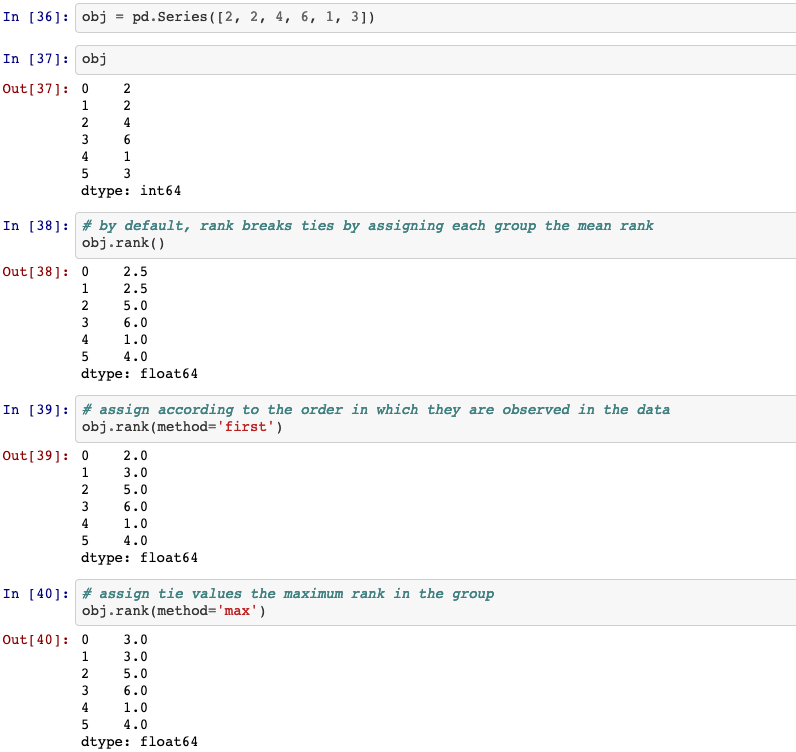## 12. is_unique

Return boolean if values in the object are unique.
Series.is_unique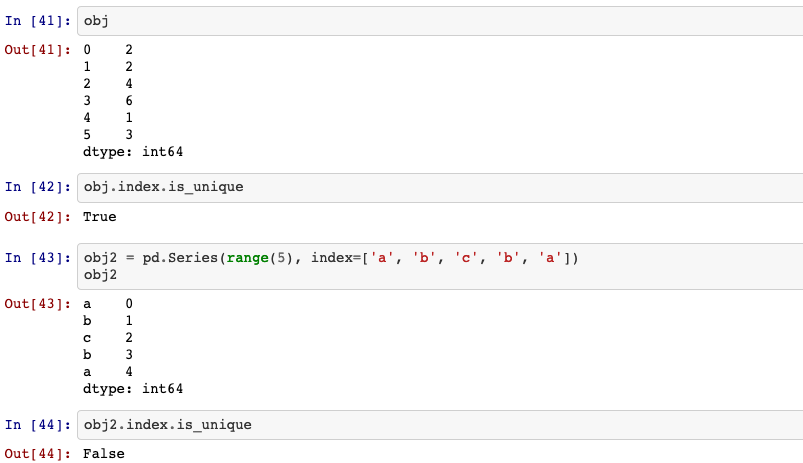## 13. isin()

Check whether values are contained in Series.
Series.isin(self, values)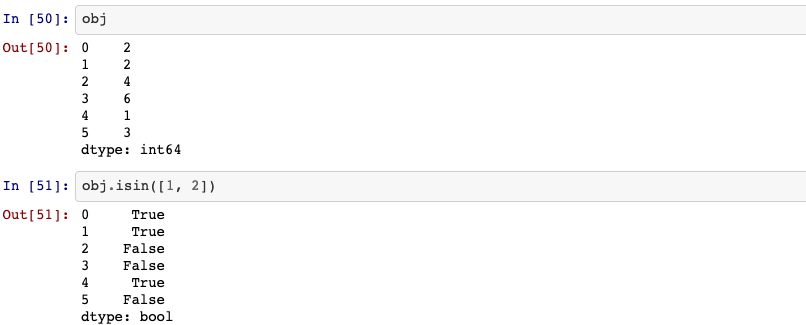## 14. Computing descriptive statistics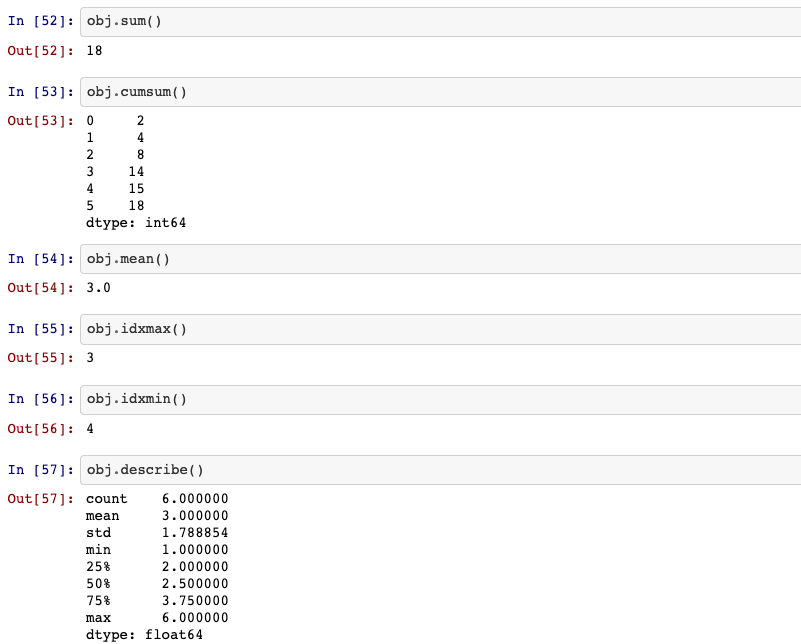## 15. unique values

Return unique values of Series object.
Series.unique(self)

Return number of unique elements in the object.
Series.nunique(self, dropna=True)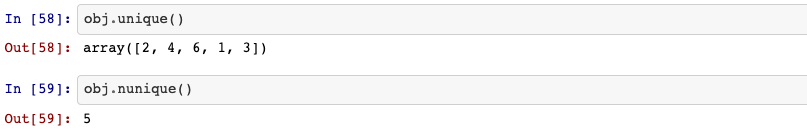## 16. value_counts

Return a Series containing counts of unique values.
Series.value_counts(self, normalize=False, sort=True, ascending=False, bins=None, dropna=True)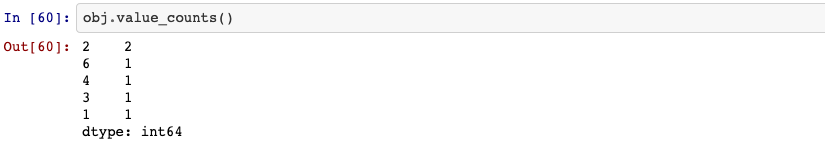## 17. Filtering out missing data

Return a new Series with missing values removed.
Series.dropna(self, axis=0, inplace=False, how=None)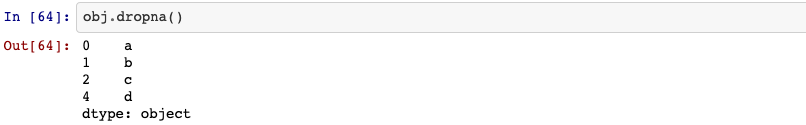Detect existing (non-missing) values.
Series.notnull(self)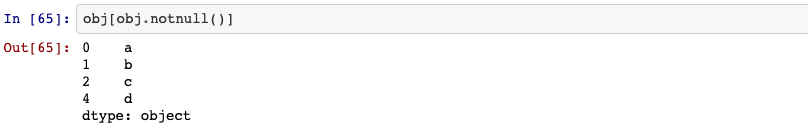## 18. Filling in missing data

Fill NA/NaN values using the specified method.
Series.fillna(self, value=None, method=None, axis=None, inplace=False, limit=None, downcast=None)

• method: {‘backfill’, ‘bfill’, ‘pad’, ‘ffill’, None}, default None. Method to use for filling holes in reindexed Series.
• pad / ffill: propagate last valid observation forward to next valid
• backfill / bfill: use next valid observation to fill gap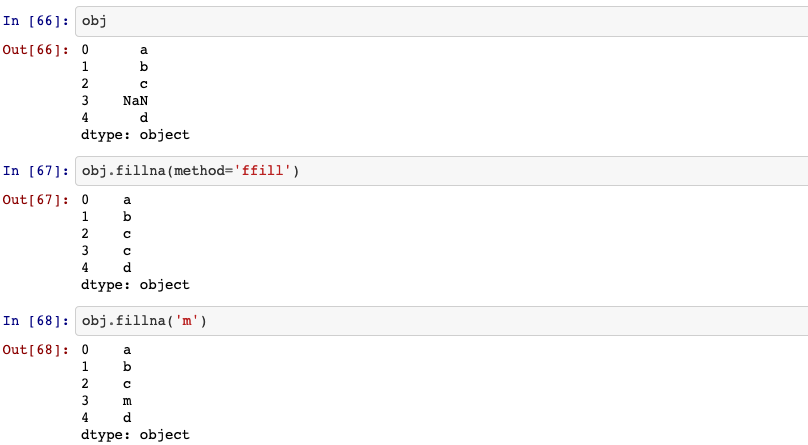## 19. Removing duplicates

Indicate duplicate Series values.
Series.duplicated(self, keep='first')

• keep: {‘first’, ‘last’, False}, default ‘first’. Method to handle dropping duplicates:
• first : Mark duplicates as True except for the first occurrence.
• last : Mark duplicates as True except for the last occurrence.
• False : Mark all duplicates as True.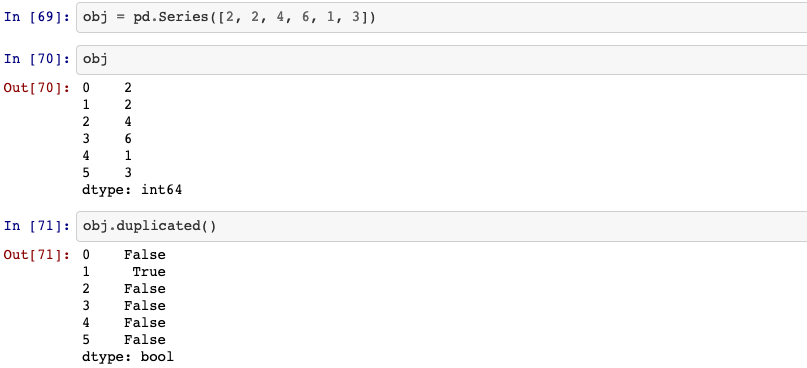Return Series with duplicate values removed.
Series.drop_duplicates(self, keep='first', inplace=False)[source]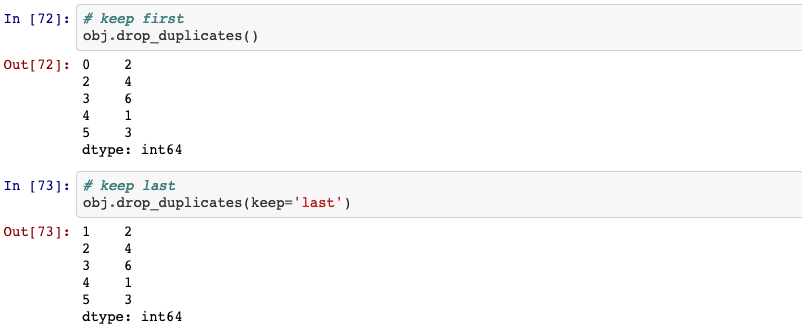## 20. map

Map values of Series according to input correspondence.
Used for substituting each value in a Series with another value, that may be derived from a function, a dict or a Series.
Series.map(self, arg, na_action=None)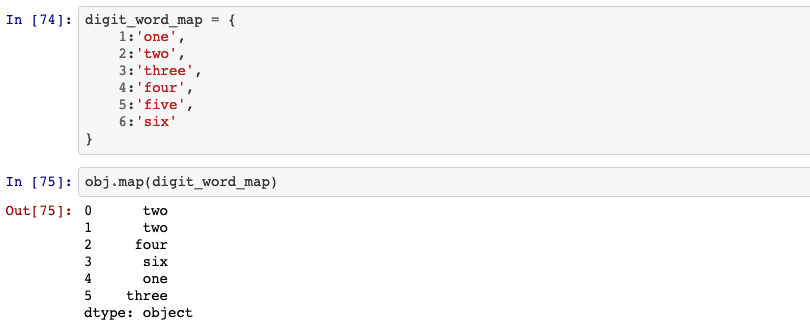## 21. apply

Invoke function on values of Series.
Can be ufunc (a NumPy function that applies to the entire Series) or a Python function that only works on single values.

Series.apply(self, func, convert_dtype=True, args=(), **kwds)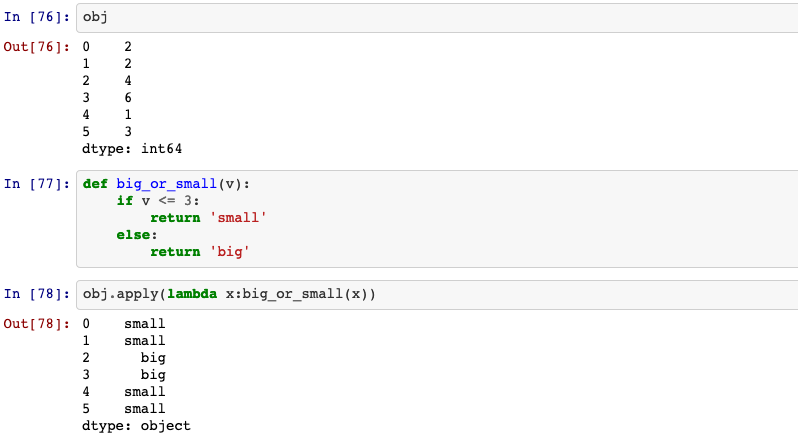## 22. replace

Replace values given in to_replace with value.
Series.replace(self, to_replace=None, value=None, inplace=False, limit=None, regex=False, method='pad')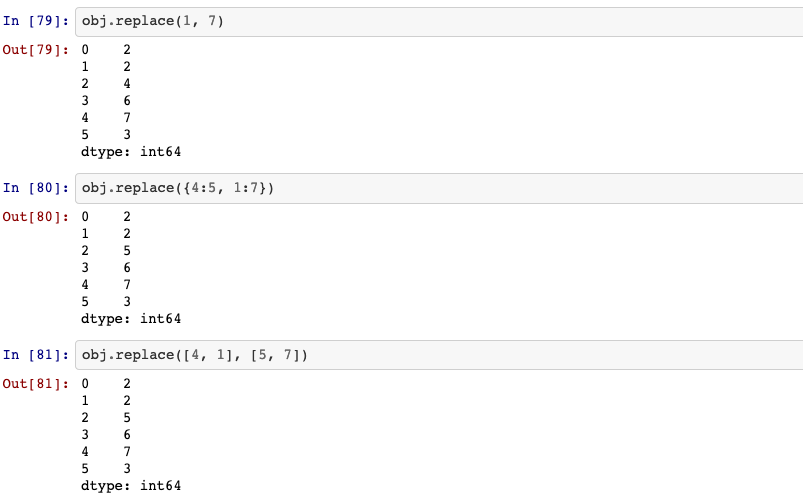## 23. concat

Concatenate pandas objects along a particular axis with optional set logic along the other axes.
pandas.concat(objs, Mapping, axis=0, join='outer', ignore_index=False, keys=None, levels=None, names=None, verify_integrity=False, sort=False, copy=True)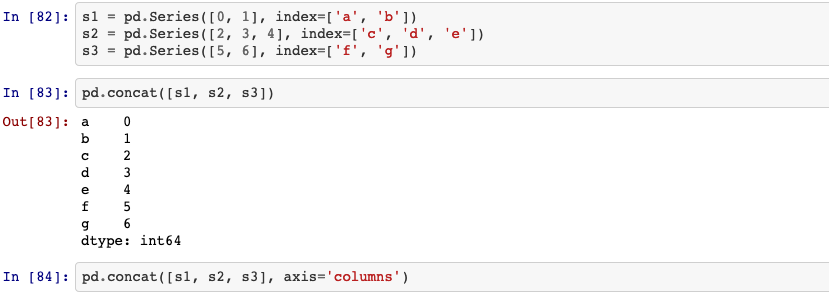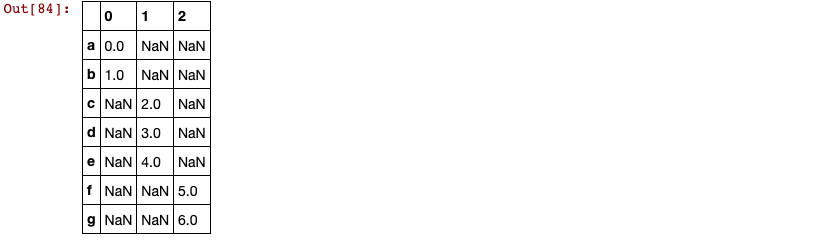## 24. numpy.where()

Return elements chosen from x or y depending on condition.
numpy.where(condition[, x, y])